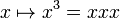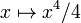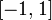# Cube function

(diff) ← Older revision | Latest revision (diff) | Newer revision → (diff)
This article is about a particular function from a subset of the real numbers to the real numbers. Information about the function, including its domain, range, and key data relating to graphing, differentiation, and integration, is presented in the article.
View a complete list of particular functions on this wiki

## Definition

This function, termed the cube function or cubing function or cube map, is the function that sends any element to its cube, i.e., to its third power. It is defined as:$\! x \mapsto x^3 = xxx$

## Key data

Item Value
default domain all real numbers, i.e., all of$\R$
range all real numbers
period not a periodic function
inverse function cube root function (both the cube function and the cube root function are one-one functions)
first derivative$x \mapsto 3x^2$, i.e., thrice the square function
second derivative$x \mapsto 6x$, i.e., six times the identity function
antiderivative$x \mapsto x^4/4$, i.e.,$1/4$ times the fourth power function
important symmetries the origin$(0,0)$ is a point of half turn symmetry
local minimum values and points of attainment there are no local minimum values because the function is increasing throughout its domain
local maximum values and points of attainment there are no local maximum values because the function is increasing throughout its domain
points of inflection (both coordinates) the origin$(0,0)$
intervals of increase/decrease and concave up/down increasing and concave down on$(-\infty,0)$.
increasing and concave up on$(0,\infty)$.

## Graph

Below is the graph of the function, with domain restricted to$[-1,1]$:

Below is the graph of the function and its derivative, with domain restricted to$[-1,1]$: